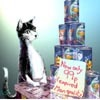#### You may also like### Summing Consecutive Numbers

15 = 7 + 8 and 10 = 1 + 2 + 3 + 4. Can you say which numbers can be expressed as the sum of two or more consecutive integers?### Human Food

Sam displays cans in 3 triangular stacks. With the same number he could make one large triangular stack or stack them all in a square based pyramid. How many cans are there how were they arranged?### Sam Again

Here is a collection of puzzles about Sam's shop sent in by club members. Perhaps you can make up more puzzles, find formulas or find general methods.

# Forgotten Number

##### Age 11 to 14Challenge Level

I have forgotten the number of the combination of the lock on my briefcase. I did have a method for remembering it...

It went as follows:

The number is equal to the sum of the cubes of its own digits.

It is a three digit number less than 500

It is the sum of consecutive factorials.

It is a triangle number.